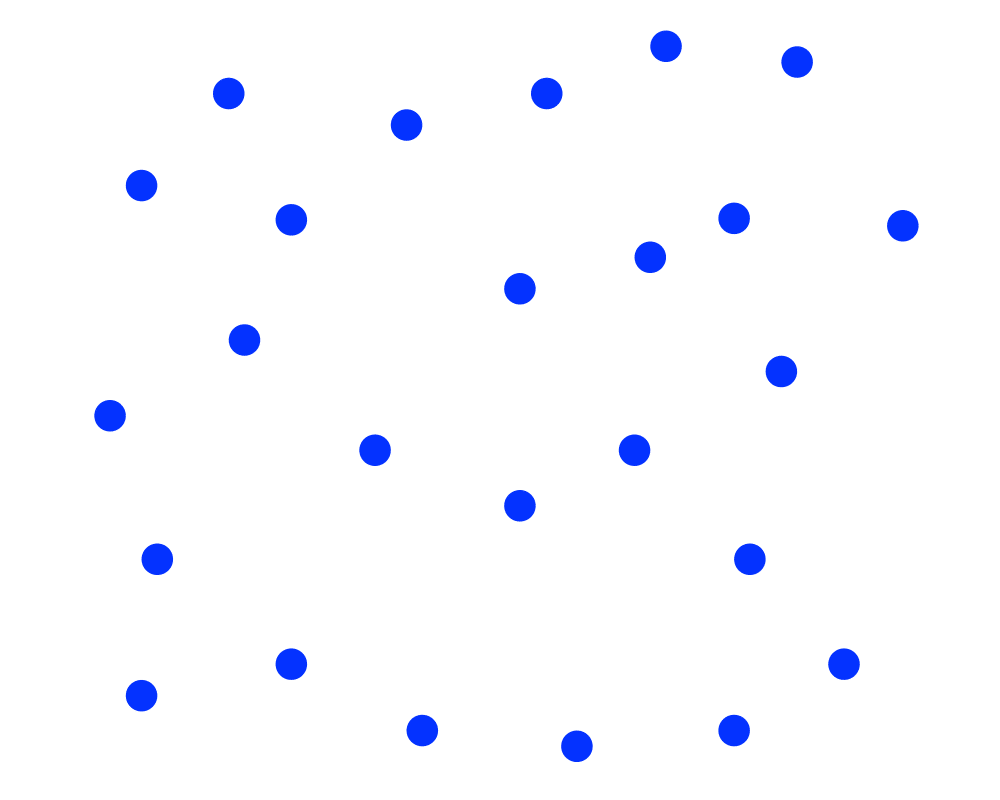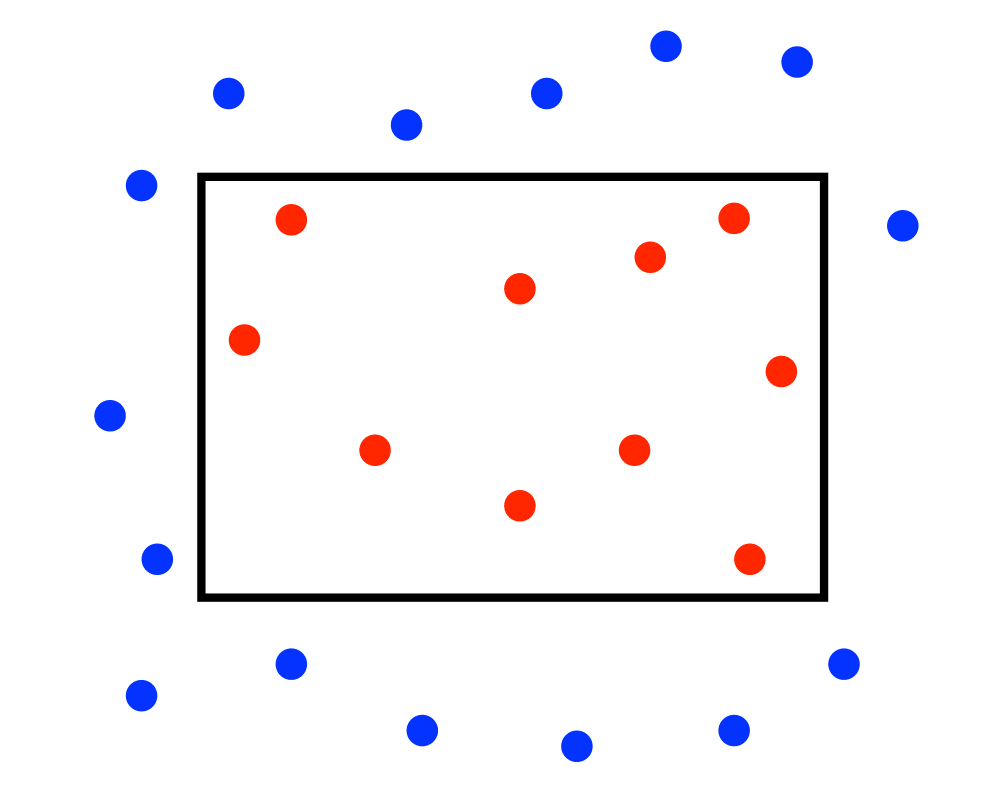# Range SearchInputOutput

Input Description: A set $$S$$ of $$n$$ points in $$E^d$$, and a query polygon $$Q$$.
Problem: Which points from $$S$$ lie within $$Q$$?

Excerpt from The Algorithm Design Manual: Range search problems arise in database and geographic information system (GIS) applications. Any data object with $$d$$ numerical fields, such as person with height, weight, and income, can be modeled as a point in $$d$$-dimensional space. A range query describes a region in space and asks for all points or the number of points in the region. For example, asking for all people with income between $0 and$10,000, with height between 6'0'' and 7'0'', and weight between 50 and 140 lbs. defines a box containing people whose body and wallets are both thin.

Go To Main Page